#Rule 29: Converting Percentages to FractionsRecall that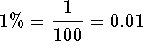. To convert a percentage to a fraction, simply convert 1% to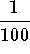. To convert a percentage to a decimal, simply convert 1% to .01.Example 1: Convert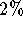to a fraction.

Answer.can be written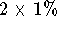which in turn can be written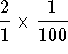. Multiply the two fractions using the multiplication rule to get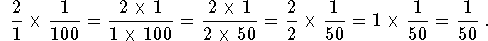Example 2: Convert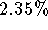to a decimal.

Answer.can be written### If you would like to review more examples, click on Example. Work the following problems and click on Answer if you want to check the answer or review the solutions.

Problem 1: Convert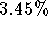to a fraction, to a decimal.

Problem 2: Convert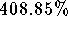to a fraction, to a decimal.

Problem 3: Convert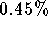to a fraction, to a decimal.[Simple Fractions] [Complex Fractions] [Compound Fractions]
[Decimals] [Percentage] [Rules]

[Algebra] [Trigonometry] [Complex Variables]S.O.S MATHematics home page

Do you need more help? Please post your question on our S.O.S. Mathematics CyberBoard.Author: Nancy Marcus